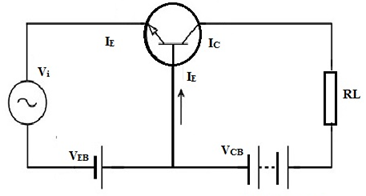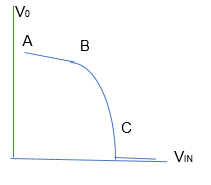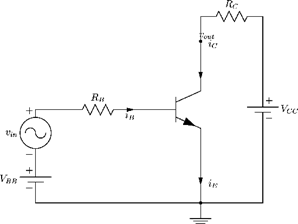# Transistor As Amplifier

One of the key characteristics of a transistor is that it can be used as an amplifier. Transistors can act as amplifiers while they are functioning in the active region or when it is correctly biased. The need for transistor as an amplifier arises when we want to increase or amplify the input signal. A transistor can take in a very small weak signal through the base junction and release the amplified signal through the collector.

Transistors amplifiers are used frequently in RF (radio frequency), OFC (optic fibre communication), audio amplification, etc. In this lesson, we will basically discuss how a transistor works as an amplifier.

## Common-Emitter Configuration

For a transistor to work as an amplifier we usually use the common-emitter configuration. The figure below shows how the transistor is set up when it is connected in a circuit as an amplifier.In the figure given above, the input is connected in forward-biased and the output is connected in reverse-biased. The input signal is applied on the base-emitter junction and the output is taken through the load in emitter-collector junction. There is also an application of DC voltage in the input circuit for amplification. Besides, a small change in signal voltage results in the change of emitter current which is mainly due to the low resistance in the input circuit.

The output is taken across the load connected on the output side. The load can be in any combination of R, L or C. The load resistance is of high value which causes a large voltage drop. Overall, the weak signal is thus amplified in the collector circuit.

However, to work as an amplifier, the transistor has to work in the active region of the output voltage versus input voltage curve as seen in the figure below.In the above graph, we have AB as the cut-off region, BC as the active region and from C we have a line parallel to X-axis, which is the saturation region.

## Gain in Power, Current and VoltageFrom the figure that is given above it is clear that on the output side, V0 = VCC– ICRC, where V0 is the output voltage, Ic is the collector current, Rc is the load resistance and Vcc is fixed voltage on the output side.

If we consider ∆V0 and ∆Vi as small changes in output and input voltages respectively, then ∆V0 / ∆Vi is called as the small-signal voltage gain, Av of the amplifier.

Therefore, ∆V0= 0 – Rc ∆IC

The gain in terms of voltage when the changes in input and output currents are observed is called Voltage gain.

Similarly in input side,

Vin = IB RB + VBE

Or

∆Vin = ∆IBRB + ∆VBE ~ ∆Vin = ∆IBRB (∆VBE <<∆IBRB)

Or,

Av = ∆V0 / ∆Vi = – Rc ∆IC / RB ∆IB = -βac Rc / RB

where βac = ∆IC / ∆IB is the AC current gain.

When there is gain in terms of current due to the changes in input and output currents is called current gain. β value can range between 20 to 500.

Power gain of an amplifier is defined as the product of current gain and voltage gain of the amplifier. It is also defined as the rate of change in output power to the change in input power.

Mathematically, Ap = βac x Av

## Use of Capacitors

We have Coupling capacitor that is used to couple the AC component and decouples the DC component contained in the input signal of the amplifier. At the output of the amplifier, the DC component will be invariantly present due to the amplification process. This is being removed by the coupling capacitor at the output and hence we will have pure AC signal being supplied to the load connected at the output.

Also Read: Capacitor Types and Capacitance

Similarly, we do have an Emitter- bypass Capacitor CE. When AC signal is applied to an amplifier circuit, the variable current will flow through resistors connected at the collector and emitter, i.e., RC and RE. This current in RE will develop a variable voltage drop across RE and provides additional negative feedback to the emitter junction.

This will result in an overall reduction of voltage gain associated with the amplifier. The capacitor CE connected across RE provides a short circuit path for the AC signal and reduce the effect of additional negative feedback due to the AC signal and a corresponding reduction in voltage gain.

## Role of Resistance

The resistors R1 and R2 form the voltage division circuit to supply the DC voltage to the base of the transistor. The resistors RC and RE control the collector and emitter currents respectively. Proper selection of these resistors helps us to control the value of the limiting currents. These resistors provide the required junction voltages between E-B, C-B, C-E and currents IE, IB and IC to work the transistor in the active region of the output characteristics.

The emitter resistor RE produces the following changes in the performance of CE amplifier:

• It causes bias stabilization
• It causes current gain to remain essentially unaltered.
• Increases the input and output impedances.
• It stabilizes the voltage gain.

## The Need for CE Configuration

We usually employ CE configuration for transistors as amplifiers because it provides large values of current gain, voltage gain and power gain. Moreover, there is a phase-shift of 180 degrees between input and output. It implies the output signal will be an inverted amplified version of the signal given in the input.

As we come to the end of the lesson, we have to know and remember that a transistor amplifier in order to function properly must have the following things;

• High input impedance.
• High gain.
• High slew rate.
• High bandwidth.
• High efficiency.
• High stability.
• High linearity.

## Solved Problems

1. Consider a CE- transistor made to work as an amplifier. The audio signal voltage across the collector resistance of 2 KΩ is 2 volts. Suppose the current amplification factor of the transistor is 100 and base resistance is 1 KΩ, determine the input signal voltage and base current?

Given RC = 2 KΩ = 2000 Ω; Vo = 2V; βac = 100; Ri = 1KΩ = 1000 Ω

Voltage gain, Av = Vo / Vi = βac R0 / Ri è 2 / Vi = 100 * (2000 / 1000) è Vi = 0.01 V.

Hence, base current, Ib = Vi / Ri= 0.01 / 1000 = 10 * 10-6 A = 10 µA.

2. 2 amplifiers are connected in a series (cascaded). The voltage gain of the first amplifier is 10 and the second has 20 voltage gain. The input signal is given as 0.01 V. Calculate the output of the AC signal?